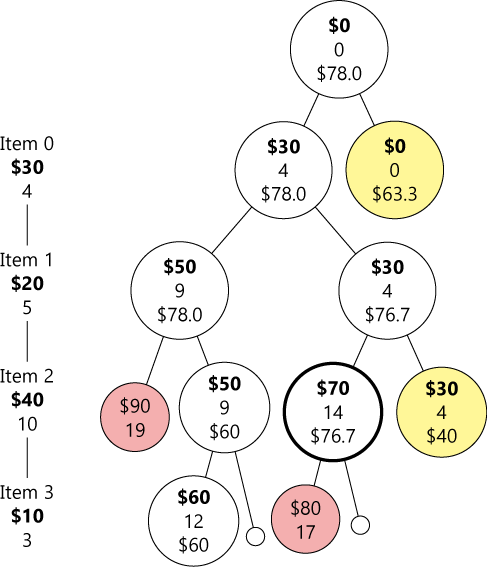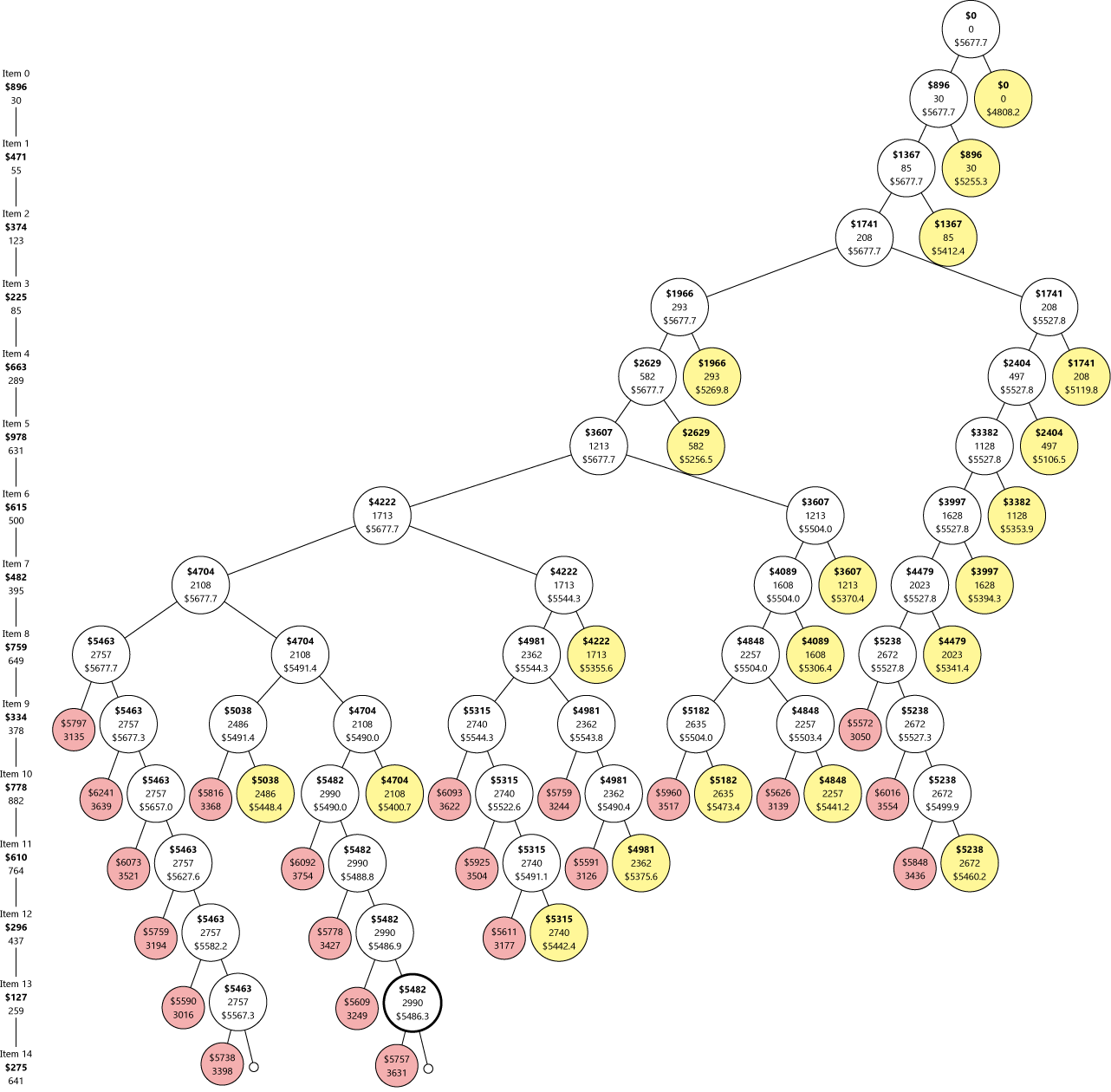# 0-1 背包问题详解 (3)

#### 哪能搞法——分支界限• 重量超限的——如果一个结点重量超限，那再往上加东西的也不用看了。
• 最下面一层的右侧打叉的结点——结果肯定和上一层一样，也不用再算一遍了。

$\tilde{W} = W_0 + \sum_{j=i+1}^{k-1} w_j$ 而总价值的上限就是
$V_{\rm bound} = \left(V_0 + \sum_{j=i+1}^{k-1} v_j \right) + \big(W – \tilde{W}\big)\cdot \frac{v_k}{w_k}$ 其中前面一项是放进去的物品总价值，后面一项是把第 $$k$$ 个物品切开后塞满包包的价值。

import sys

def knapsack(vw, limit):

def bound(v, w, j):
if j >= len(vw) or w > limit:
return -1
else:
while j < len(vw) and w + vw[j] <= limit:
v, w, j = v + vw[j], w + vw[j], j + 1
if j < len(vw):
v += (limit - w) * vw[j] / (vw[j] * 1.0)
return v

def traverse(v, w, j):
nonlocal maxValue
if bound(v, w, j) >= maxValue: # promising
if w + vw[j] <= limit:  # w/ j
maxValue = max(maxValue, v + vw[j])
traverse(v + vw[j], w + vw[j], j + 1)
if j < len(vw) - 1:        # w/o j
traverse(v, w, j + 1)
return

maxValue = 0
traverse(0, 0, 0)
return maxValue

if __name__ == "__main__":
with open(sys.argv if len(sys.argv) > 1 else sys.exit(1)) as f:
limit, n = map(int, f.readline().split())
vw = []                   # value, weight, value density
for ln in f.readlines():
vl, wl = tuple(map(int, ln.split()))
vw.append([vl, wl, vl / (wl * 1.0)])

print(knapsack(sorted(vw, key=lambda x : x, reverse=True), limit))RuntimeError: maximum recursion depth exceeded while calling a Python object

import thread
...
def main():
...

if __name__ == "__main__":
threading.stack_size(67108864) # 64MB stack
sys.setrecursionlimit(20000)
thread = threading.Thread(target=main)
thread.start()

def knapsack(vw, limit):

def bound(v, w, j):
...

maxValue = 0
stack = [[0, 0, 0]] # value, weight, index

while stack:
v, w, j = stack.pop()
if bound(v, w, j) >= maxValue:
if j < len(vw) - 1:
stack.append([v, w, j + 1])
if w + vw[j] <= limit:
maxValue = max(maxValue, v + vw[j])
stack.append([v + vw[j], w + vw[j], j + 1])

return maxValue